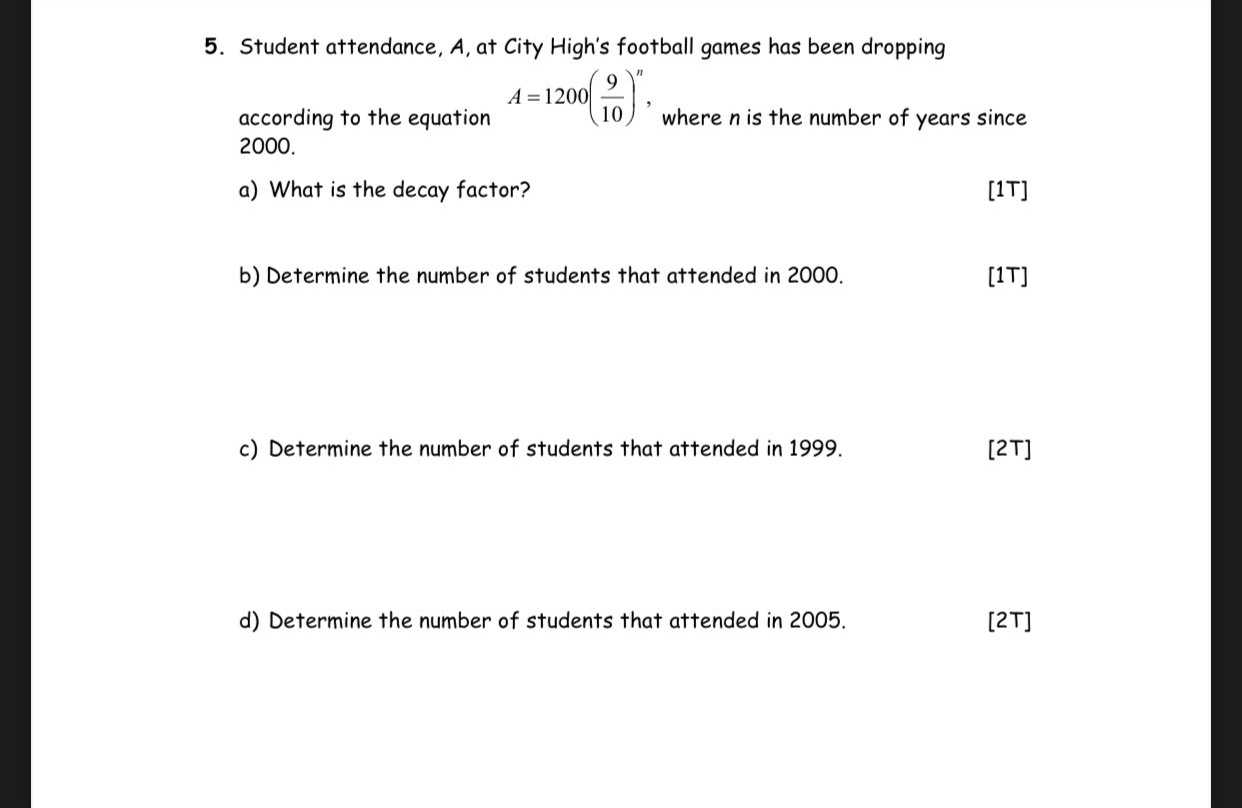### ¿Todavía tienes preguntas de matemáticas?

Pregunte a nuestros tutores expertos
Algebra
Pregunta5. Student attendance, $$A ,$$ at City High's football games has been dropping according to the equation $$A = 1200 ( \frac { 9 } { 10 } ) ^ { n } ,$$ where $$n$$ is the number of years since

$$2000 .$$

a) What is the decay factor?

b) Determine the number of students that attended in $$2000 .$$

c) Determine the number of students that attended in $$1999 .$$

d) Determine the number of students that attended in $$2005 .$$

5.

a)

Decay factor is = 1 - 9 / 10 = 1 / 10

b)

When n = 0,

$$A= 1200(\frac{9}{10})^0\\= 1200$$

c)

When n = -1,

$$A = 1200(\frac{9}{10})^{- 1}\\A = 1200 (\frac{10}{9}) \approx 1333$$

d)

When n = 5,

$$A = 1200(\frac{9}{10})^5\\ = 708$$

Solución
View full explanation on CameraMath App.